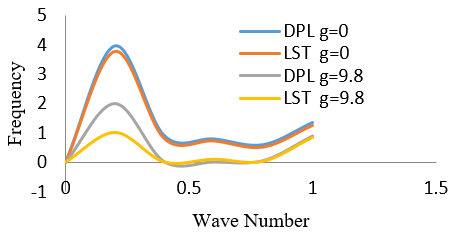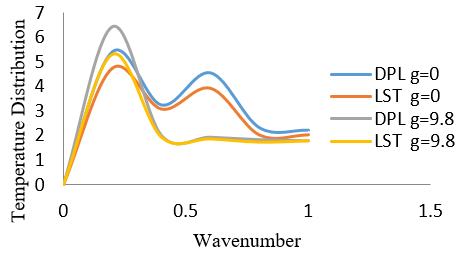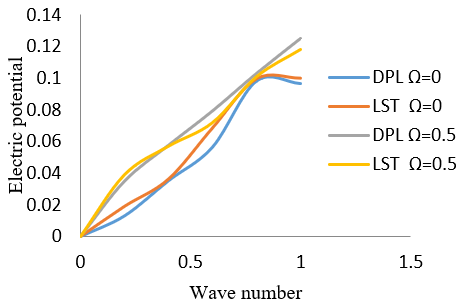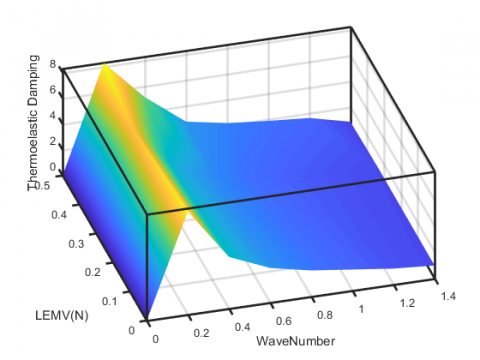# Modeling and Assessment of Rotation and Gravity in a Piezoelectric Viscothermoelastic Multilayered Composite LEMV / CFRP Cylinder

Modeling and Assessment of Rotation and Gravity in a Piezoelectric Viscothermoelastic Multilayered Composite LEMV / CFRP Cylinder
Department of Mathematics, Karunya Institute of Technology and Sciences, Coimbatore 641114, India

Departments of Mathematics, V.S.B College of Engineering, Karur 639111, India

Departments of Mathematics, Government Arts College, Udumalpet 642126, India

Corresponding Author Email:
selvam1729@gmail.com
Page:
611-616
|
DOI:
https://doi.org/10.18280/mmep.080414
6 January 2020
| |
Accepted:
28 June 2021
| | Citation

OPEN ACCESS

Abstract:

A mathematical model is developed to analysis the effects of gravitational force and rotation in a composite multilayered hollow cylinder which contain inner and outer piezo-thermoelasticity layers bonded by Linear Elastic Material with Voids (LEMV) is performed within the frame of dual-phase-lag model. The equation of displacement components, temperature, and electric are obtained using linear theory of elasticity. The dispersion equations are derived based on traction free boundary conditions and are numerically examined for CdSe material. The enumerated frequency, thermal and electrical nature against wave number is presented graphically. Adhesive layer LEMV is compared with Carbon Fiber Reinforced Polymer (CFRP) in the presence of gravity and rotation.

Keywords:

thermoelascity, multilayered cylinders, LEMV, CFRP, dual phase lagging model

1. Introduction

Piezoelectric materials are ordinarily utilized for savvy structure applications because of their direct and converse piezoelectric impacts which enable them to be used as the both actuators and sensors. The structure and development of piezoelectric whirligigs and other pivoting sensors have significant applications in innovation. The investigation of the impacts of turn on the proliferation of waves in piezo-thermoelastic cylinder has been broadly examined in the previous two decades.

The generalized lagging performance in solids beneath high-rate heating is derived by precise correlation with the hyperbolic two-step model. The ballistic performance of heat transport in the electron gas is established to be captured by the second-order effect of the phase lag of the heat flux vector. In contrast to the parabolic two-step model, the ballistic behavior results in a sharp wave front in the history of heat propagation. Lord and Shulman  at first investigation the generalized dynamical hypothesis of thermoelasticity. Singh et al.  study proliferation of Rayleigh wave in two temperature dual phase lag thermoelasticity. Green and Lindsay  explored different parts of thermoelasticity. Othman et al.  examined impact of magnetic field on generalized piezo-thermoelastic rotating medium with two relaxation times. Assessment of the fundamental properties of thermomechanics, by Green and Naghdi . Mindlin  determined the conditions of high recurrence vibrations of thermo-piezo-electric plate. Green and Naghdi  talked about damped heat waves in a elastic solid. Abou-Dina et al.  figure a model for nonlinear thermo-electroelasticity in broadened thermo-electroelasticity in expanded thermoelasticity. Green and Naghdi  quickly clarify thermoelasticity without vitality scattering. Abo-Dahab  examined proliferation of Stoneley waves in magnetothermoelastic materials with voids and two unwinding times. Abd-Alla et al.  considered propagation of Rayleigh waves in magneto-thermo-versatile half–space of a homogeneous orthotropic material under the impact of the rotating, starting pressure and gravity field. Soderkvist  played out a thought for smaller scale machined gyroscopes actuators. Achenbach  researched wave proliferation in elastic solids. Impact of magnetic field on poroelastic bone model for inward rebuilding by Abd-Alla and Abo-Dahab . Othman and Lotfy  talked about the impact of magnetic field and rotate of the 2-D issue of a fiber-fortified thermoelastic under three hypotheses with impact of gravity. Samal and Chattaraj  detail another advancement for surface wave proliferation in fiber reinforced anisotropic elastic layer between fluid immersed permeable half space and uniform fluid layer. Elnaggar and Abd-Alla  researches rayleigh waves in magneto-thermo-microelastic half-space under starting pressure. Paul and Raman  found wave engendering in a pyroelectric cylinder of arbitrary cross segment with a round cylindrical cavity. Paul and Nelson  plan ideas of axisymmetric vibration of piezocomposite hollow circular cylinder. Puri and Cowin  found plane waves in direct elastic materials with voids. The old style pressure vessal issues for direct elastic material with voids talked about by Cowin and Puri . Ponnusamy  examined wave proliferation in a piezoelectric solid bar of circular cross-section immersed in fluid. Impact of rotation on generalized thermo-viscoelastic Rayleigh-Lamb waves by Sharma and Othman . Assaf et al.  explored vibration and acoustic reaction of damped sandwich plates drenched in a light or heavy fluid. Tzou  discovered the macro to microscale heat transfer through the lagging behavior. Hobiny and Abbas . generalized thermoelastic interaction in a two-dimensional porous medium under dual phase lag model. Mahesh and Selvamani  formulate mathematical model for bending analysis of generalized thermoelastic waves in a multilayered cylinder using theory of dual phase lagging.

The present paper, DPL theory is used to study the influence of gravity and rotation on piezo-thermoelastic cylinder. The equation of displacement components, temperature and electric are obtained using linear theory of elasticity. The computed non-dimensional frequency is presented in the form of dispersion curves against various physical variables. Adhesive layer LEMV is compared with Carbon Fiber Reinforced Polymer (CFRP) in presence of gravity and rotation. The whole papers layout are given below:

1. PROBLEM FORMULATION
2. EQUATION OF MOTION FOR LINEAR ELASTIC MATERIALS WITH VOIDS LEMV
3. BOUNDARY CONDITIONS AND FREQUENCY EQUATIONS
4. NUMERICAL DISCUSSION
5. CONCLUSIONS
2. Problem Formulation

We deal with a homogeneous transversely isotropous thermally and electrically conducting composite multilayered hollow cylinder of limitless length with constant temperature T_0 in an unvaried state at the beginning. Cylinder rotating uniformly with an angular rate a couple of fastened axis in area with angular velocity Ω. In cylindrical coordinates (r, θ, z), the equations of motion within the absence of body force and as well as the outcome and centripetal forces are :

\begin{aligned} c_{11}\left(u_{, r r}^{l}+r^{-1} u_{, r}^{l}\right.&\left.+r^{-2} u^{l}\right)+c_{13} w_{, r z}^{l} \\ &+c_{44}\left(u_{, z z}^{l}+w_{, r z}^{l}\right) \\ &+\left(e_{15}+e_{31}\right) \varphi_{, r z}^{l}+\rho g w_{, r} \\ &-\beta_{1} T_{, r}^{l}+\rho\left(\Omega^{2} u+2 \Omega w_{, t}\right) \\ &=\rho u_{t t} \end{aligned}   (1a)

\begin{aligned} \left(c_{44}+c_{13}\right)\left(u^{l}, r z\right.&\left.+r^{-1} u_{, z}^{l}\right)+c_{33}\left(w_{, z z}^{l}\right) \\ &+c_{44}\left(w_{, r r}^{l}+r^{-1} w^{l}, r\right) \\ &+e_{15}\left(\varphi_{, r r}^{l}+r^{-1} \varphi_{, r}\right)-\rho g u_{r} \\ &-\beta_{3} T_{, r}^{l}+\rho\left(\Omega^{2} w+2 \Omega u_{, t}\right) \\ &=\rho w_{t t} \end{aligned}   (1b)

$\begin{gathered} r^{-1} e_{15}\left(w_{, r r}^{l}+u_{, r z}^{l}\right)+\varepsilon_{11} \varphi_{,r}^{l}+e_{13} u_{, r z}^{l} \\ \quad+r^{-1}\left(u_{, r}^{l}+v_{, \theta z}^{l}\right)+e_{33} w,_{z z}^{l} \\ \quad+\varepsilon_{33} \varphi,_{z}^{l}+p_{3} T=0 \end{gathered}$   (1c)

\begin{aligned} k_{1} T_{, r r}+k_{3} T_{, z z}+& \tau_{\theta}\left(k_{1} T_{, r r}+k_{3} T_{, z z}\right)_{, t} \\ &=\left(1+\tau_{q} \frac{\partial}{\partial t}\right)\left[\rho C_{T} T\right.\\ &\left.+T_{0}\left(\beta_{1} u,_{r}+\beta_{3} w_{, z}-p_{3} \phi_{, z}\right)\right] \end{aligned}   (1d)

The solution of Eq. (1) is considered in the form .

$\begin{gathered} u^{l}=U^{l}, r \exp \{i(k z+p t)\} \\ w^{l}=\left(\frac{i}{h}\right) W^{l} \exp \{i(k z+p t)\} \\ \varphi^{l}=\left(i c_{44} / a e_{33}\right) E^{l} e^{i(k z+p t)} \\ T^{l}=\left(\frac{c_{44}}{\beta_{3}}\right)\left(\frac{T^{l}}{h^{2}}\right) \exp \{i(k z+p t) \end{gathered}$     (2)

where, $u^{l}, w^{l}, \varphi^{l}, T^{l}$ are displacement potentials, k denotes wave number, p denotes angular frequency and $i=\sqrt{-1}$. We introduce the non dimensional quantities $x=\frac{r}{a}, \varepsilon=k a, c=\rho p$, ‘a’ denotes geometrical parameter of the composite hollow cylinder. $\bar{c}_{11}={ }^{c_{11}} / c_{44}, \bar{c}_{13}={ }^{c_{13}} / c_{44}$, $\bar{c}_{33}={ }^{c_{33}} / c_{44}, \bar{c}_{66}={ }^{c_{66}} / c_{44}, \bar{\beta}=\beta_{1} / \beta_{3}$, $\bar{k}_{i}=\frac{\left(\rho c_{\quad44}\right)\quad^{\frac{1}{2}}}{\beta_{3}^{2}\quad T_{0}\quad a\quad \Omega}$. Substituting the Eq. (2) in Eq. (1) we obtain,

$\begin{gathered} \left.\overline{[c}_{11}^{l} \nabla^{2}+\varepsilon^{2}-(c a)^{2}\right] U^{l}-\varepsilon\left(1+\bar{c}_{13}^{l}\right) W^{l}+\varepsilon\left(\bar{e}_{15}^{l}+\right. \\ \left.\bar{e}_{31}^{l}\right) E^{l}-\bar{\beta} T^{l}=0 \\ \left.\varepsilon\left(1+\bar{c}_{13}^{l}\right) \nabla^{2} U^{l}+\overline{[} c_{44}^{l} \nabla^{2}+\varepsilon^{2} \bar{c}_{33}^{l}-(c a)\right] W^{l}+\left(\bar{e}_{15} \nabla^{2}+\right. \\ \left.A_{6}\right) \bar{c}_{44} E^{l}+\varepsilon T^{l}=0 \\ A_{3} \nabla^{2} U^{l}+\left[\bar{e}_{15} \nabla^{2}+\varepsilon^{2}\right] W^{l}+\left\lceil-\frac{K_{33}}{K} \nabla^{2}+\bar{K}_{33}^{2} \varepsilon^{2}\right] E^{l} \\ -p^{l} \varepsilon T^{l}=0 \\ \begin{array}{c} \left.\nabla^{2} U^{l}-M W^{l}+M_{3} E^{l}+\mid \frac{K \nabla^{2}}{\beta^{*} a^{2}}\left(1+\tau_{t}\right)-M_{1}-M_{2}\right] T^{l} \\ =0 \end{array} \end{gathered}$

The above relation reformulated as follows:

$\left(\begin{array}{cccc} \bar{c}_{11}^{l} \nabla^{2}+A_{1} & -A_{2} & A_{3} & -A_{4} \\ A_{2} \nabla^{2} & \bar{c}_{44}^{l} \nabla^{2}+A_{5} & \left(\bar{e}_{15} \nabla^{2}+A_{6}\right) \bar{c}_{44} & A_{7} \\ A_{3} \nabla^{2} & \bar{e}_{15} \nabla^{2}+A_{6} & -\frac{K_{33}}{K} \nabla^{2}+A_{8} & -A_{9} \\ \iota \nabla^{2} & -M & M_{3} & \frac{K \nabla^{2}}{\beta^{*} a^{2}}\left(1+\tau_{t}\right)-M_{1}-M_{2} \end{array}\right)\left(U^{l}, W^{l}, E^{l}, T^{l}\right)^{T}=0$   (3)

where, $\nabla^{2}=\frac{\partial^{2}}{\partial x^{2}}+\frac{1}{x} \frac{\partial}{\partial x}, A_{1}=\varepsilon^{2}-(c a)^{2}, A_{2}=\varepsilon\left(1+\bar{c}_{13}^{l}\right)$,

\begin{aligned} A_{3} &=\varepsilon\left(\bar{e}_{15}^{l}+\bar{e}_{31}^{l}\right), A_{4}=\bar{\beta}, A_{5}=\varepsilon^{2} \bar{c}_{33}^{l}-(c a), A_{6}=\\ \varepsilon^{2}, A_{7} &=\varepsilon, A_{8}=\bar{K}_{33}^{2} \varepsilon^{2}, M_{3}=-\frac{i p_{3} \beta^{*} T_{0} z k p}{e_{33} a^{2}}, M=\frac{\tau_{q} p^{2} i a}{c_{44}} \\ M_{1} &=\frac{k^{2}}{\beta^{*}}\left(1+i p \tau_{t}\right), M_{2}=\frac{\rho^{l} c_{v} i p}{\beta^{*}}\left(1-\tau_{q} p\right), A_{9}=p^{l} \varepsilon \\ \iota=\frac{\beta^{*} T_{0} z}{c_{44}} \end{aligned}

Eq. (3), reformulated as the following form

$\left(A \nabla^{8}+B \nabla^{6}+C \nabla^{4}+D \nabla^{2}+E\right)\left(U^{l} W^{l} E^{l} T^{l}\right)^{T}=0$     (4)

The solution of Eq. (4) is obtained as

$\begin{array}{r} U^{l}=\sum_{i=1}^{4}\left[A_{j} J_{n}\left(\alpha_{j} x\right)+B_{j} y_{n}\left(\alpha_{j} x\right)\right] \\ W^{l}=\sum_{i=1}^{4} a_{j}^{l}\left[A_{j} J_{n}\left(\alpha_{j} x\right)+B_{j} y_{n}\left(\alpha_{j} x\right)\right] \\ E^{l}=\sum_{i=1}^{4} b_{j}^{l}\left[A_{j} J_{n}\left(\alpha_{j} x\right)+B_{j} y_{n}\left(\alpha_{j} x\right)\right] \\ T^{l}=\sum_{j=1}^{4} c_{j}^{l}\left[A_{j} J_{n}\left(\alpha_{j} x\right)+B_{j} y_{n}\left(\alpha_{j} x\right)\right] \end{array}$    (5)

Here $\left(\alpha_{i}^{l} a x\right)>0$, for (i=1,2,3,4) are the zeros of algebraic equation.

$\begin{gathered} \left(A\left(\alpha^{l} a\right)^{8}+B\left(\alpha^{l} j a\right)^{6}+C\left(\alpha^{l} a\right)^{4}+D\left(\alpha^{l} a\right)^{2}+\right. \\ E)\left(U^{l}, W^{l}, E^{l}, T^{l}\right)=0 \end{gathered}$    (6)

The constants $a_{j}^{l}, e_{j}^{l} \text { and } c_{j}^{l}$ can be evaluated using the following relations:

$\begin{gathered} \left.\overline{[c}_{11}^{l} \nabla^{2}+\varepsilon^{2}-(c a)^{2}\right]-\varepsilon\left(1+\bar{c}_{13}^{l}\right) a_{j}^{l}+\varepsilon\left(\bar{e}_{15}^{-l}+\bar{e}_{31}^{l}\right) e_{i}^{l} \\ -\bar{\beta} c_{j}^{l}=0 \\ \left.\varepsilon\left(1+\bar{c}_{13}^{l}\right) \nabla^{2}+\bar{c}_{44}^{l} \nabla^{2}+\varepsilon^{2} \bar{c}_{33}^{l}-(c a)\right] a_{j}^{l}+\left(\bar{e}_{15} \nabla^{2}+\right. \\ \left.A_{6}\right) \bar{c}_{44} b_{j}^{l}+\varepsilon c_{i}^{l}=0 \\ A_{3} \nabla^{2}+\left[\bar{e}_{15} \nabla^{2}+\varepsilon^{2}\right] a_{j}^{l}+\left[-\frac{K_{33}}{K} \nabla^{2}+\bar{K}_{33}^{2} \varepsilon^{2}\right] b_{j}^{l}-p^{l} \varepsilon c_{j}^{l} \\ =0 \\ \iota \nabla^{2}-M a_{j}^{l}+M_{3} b_{j}^{l}+\left[\frac{K \nabla^{2}}{\beta^{*} a^{2}}\left(1+\tau_{t}\right)-M_{1}-M_{2}\right] c_{j}^{l}=0 \end{gathered}$

3. Equation of Motion for Linear Elastic Materials with Voids LEMV

The equations of motion for isotropic LEMV materials are given as :

$\begin{gathered} (\lambda+2 \mu)\left(u,_{r r}+r^{-1} u_{, r}-r^{-2} u\right)+\mu u,_{z z} \\ +(\lambda+\mu) w_{, z z}+\beta E_{, r}=\rho u_{t t} \\ (\lambda+\mu)\left(u,_{r z}+r^{-1} u_{, z}\right)+\mu\left(w_{, r r}+r^{-1} w_{, r}\right) \\ +(\lambda+2 \mu) w_{, z z}+\beta E_{, z}=\rho w_{, t t} \\ -\beta\left(u,_{r}+r^{-1} u\right)-\beta w_{, z}+\alpha\left(E,_{r r}+r^{-1} E_{, r}+\phi_{, z z}\right) \\ -\delta k E,_{t t}-\omega E_{, t}-\xi E=0 \end{gathered}$     (7)

The stress in the LEMV core materials are,

$\begin{gathered} \sigma_{, r r}=(\lambda+2 \mu) u,_{r}+\lambda r^{-1} u+\lambda w_{, z}+\beta \phi \\ \sigma_{r z}=\mu\left(u,_{t}+w_{, r}\right) \end{gathered}$

The solution of for Eq. (7) is taken as

$\begin{gathered} u=U_{, r} \operatorname{expi}(k z+p t) \\ w=\left(\frac{i}{h}\right) W \operatorname{expi}(k z+p t) \\ E=\left(\frac{1}{h^{2}}\right) E \operatorname{expi}(k z+p t) \end{gathered}$     (8)

The above solution in (7) and nondimensionl variables x and $\varepsilon$, equation can be reduced as

$\begin{array}{ccc} \left|\begin{array}{ccc} (\lambda+2 \mu) \nabla^{2}+M_{1} & -M_{2} & M_{3} \\ M_{2} \nabla^{2} & \bar{\mu} \nabla^{2}+M_{4} & M_{5} \\ -M_{3} \nabla^{2} & M_{5} & \alpha \nabla^{2}+M_{6} \end{array}\right|(U, W, E) \\ =0 \end{array}$     (9)

where, $\nabla^{2}=\frac{\partial^{2}}{\partial x^{2}}+\frac{1}{x} \frac{\partial}{\partial x}, M_{1}=\frac{\rho}{\rho^{1}}(c h)^{2}-\bar{\mu} \varepsilon^{2}, M_{2}=(\bar{\lambda}+\bar{\mu}) \varepsilon$, $M_{3}=\bar{\beta}, M_{4}=\frac{\rho}{\rho^{1}}(c h)^{2}-(\bar{\lambda}+\bar{\mu}) \varepsilon^{2}, M_{5}=\bar{\beta} \varepsilon$, $M_{6}=\frac{\rho}{\rho^{1}}(c h)^{2} \bar{k}-\bar{\alpha} \varepsilon^{2}-i \bar{\omega}(c h)-\bar{\xi}$

The Eq. (9) can be specified as,

$\left(\nabla^{6}+P \nabla^{4}+Q \nabla^{2}+R\right)(U, W, E)=0$     (10)

Thus, the solution of Eq. (10) is as follows,

$\begin{gathered} U=\sum_{j=1}^{3}\left[A_{j} J_{0}\left(\alpha_{j} x\right)+B_{j} y_{0}\left(\alpha_{j} x\right)\right], \\ W=\sum_{j=1}^{3} a_{j}\left[A_{j} J_{0}\left(\alpha_{j} x\right)+B_{j} y_{0}\left(\alpha_{j} x\right)\right], \\ E=\sum_{i=1}^{3} b_{j}\left[A_{j} J_{0}\left(\alpha_{j} x\right)+B_{j} y_{0}\left(\alpha_{j} x\right)\right], \end{gathered}$

$\left(\alpha_{j} x\right)^{2}$  are zeros of the equation when replacing.

$\nabla^{2}=-\left(\alpha_{j} x\right)^{2}$. The arbitrary constant $a_{j} \text { and } b_{j}$ are obtained from:

\begin{aligned} &M_{2} \nabla^{2}+\left(\bar{\mu} \nabla^{2}+M_{4}\right) a_{j}+M_{5} b_{j}=0, \\ &-M_{3} \nabla^{2}+M_{5} a_{j}+\left(\alpha \nabla^{2}+M_{6}\right) b_{j}=0 \end{aligned}

For the governing equation of CFRP core material, we assume void volume fraction E=0, and the lame’s constants as $\lambda=c_{12}, \mu=\frac{c_{11}-c_{12}}{2}$  in the Eq. (7).

4. Boundary Conditions and Frequency Equations

The frequency equations can be obtained for the following boundary condition.

• On the traction free inner and outer surface $\sigma_{r r}^{l}=\sigma_{r z}^{l}=E^{l}=T^{l}=0 \text { with } l=1,3$.
• At the interface $\sigma_{r r}^{l}=\sigma_{r r} ; \sigma_{r z}^{l}=\sigma_{r z} ; E^{l}=0 ; T^{l}=0 ; D^{l}=0$.

Substituting the above boundary condition, we obtained as a 22×22 determinant equation.

$\begin{gathered} \left|\left(Y_{i j}\right)\right|=0,(i, j=1,2,3, \ldots .22) \\ \text { At } x=x_{0} \text { where } j=1,2,3,4 \\ Y_{1 j}=2 \bar{c}_{66}\left(\frac{\alpha_{j}^{1}}{x_{0}}\right) J_{1}\left(\alpha^{1}{ }_{j} x_{0}\right) \\ -\left[\left(\alpha^{1}{ }_{j} a\right)^{2} \bar{c}_{11}+\zeta \bar{c}_{13} a_{j}^{l}+\bar{e}_{31} \zeta b_{j}^{l}\right. \\ \left.+\bar{\beta} c_{j}^{l}\right] J_{0}\left(\alpha^{1}{ }_{j} a x_{0}\right) \\ Y_{2 j}=\left(\zeta+a_{j}^{1}+\bar{e}_{15} b_{j}^{1}\right)\left(\alpha_{j}^{1}\right) J_{1}\left(\alpha^{1}{ }_{j} x_{0}\right) \\ Y_{3 j}=b_{j}^{1} J_{0}\left(\alpha^{1}{ }_{j} x_{0}\right) \\ Y_{4 j}=\frac{h_{j}^{1}}{x_{0}} J_{0}\left(\alpha^{1}{ }_{j} x_{0}\right)-\left(\alpha_{j}^{1}\right) J_{1}\left(\alpha^{1}{ }_{j} x_{0}\right) \end{gathered}$

And the other nonzero elements $Y_{1, j+4} , Y_{2, j+4} , Y_{3, j+4}$  and $Y_{4, j+4}$  are obtained by replacing $J_{0} \text { by } J_{1} \text { and } Y_{0} \text { by } Y_{1}$.

$\begin{gathered} \text { At } x=x_{1} \\ Y_{5 j}=2 \bar{c}_{66}\left(\frac{\alpha_{j}^{1}}{x_{1}}\right) J_{1}\left(\alpha^{1}{ }_{j} x_{1}\right) \\-\left[\left(\alpha^{1}{ }_{j} a\right)^{2} \bar{c}_{11}+\zeta \bar{c}_{13} a_{j}^{l}+\bar{e}_{31} \zeta b_{j}^{l}\right. \\\left.+\bar{\beta} c^{l}{ }_{j}\right] J_{0}\left(\alpha^{1}{ }_{j} a x_{1}\right) \\ Y_{5, j+8}=-\left[2 \bar{\mu}\left(\frac{\alpha_{j}}{x_{1}}\right) J_{1}\left(\alpha x_{1}\right)\right. \\+\left\{-(\bar{\lambda}+\bar{\mu})\left(\alpha_{j}\right)^{2}+\bar{\beta} b_{j}\right. \\\left.-\bar{\lambda} \zeta a_{j}\right\} J_{0}\left(\alpha_{j} x_{1}\right) \\ Y_{6 j}=\left(\zeta+a_{j}^{1}+\bar{e}_{15} b_{j}^{1}\right)\left(\alpha_{j}^{1}\right) J_{1}\left(\alpha^{1}{ }_{j} a x_{1}\right) \\ Y_{6, j+8}=-\bar{\mu}\left(\zeta+a_{j}\right)\left(\alpha_{j}\right) J_{1}\left(\alpha_{j} x_{1}\right) \\ Y_{7 j}=\left(\alpha_{j}^{l}\right) J_{1}\left(\alpha_{j}^{l} x_{1}\right) \\ Y_{7, j+8}=-\left(\alpha_{j}\right) J_{1}\left(\alpha^{l} x_{1}\right) \\ Y_{8 j}=a_{j}^{l} J_{0}\left(\alpha_{j}^{l} x_{1}\right) \\ Y_{8, j+8}=-a_{j}^{l} J_{0}\left(\alpha_{j}^{l} x_{1}\right) \\ Y_{9 j}=b_{j}^{l} J_{0}\left(\alpha_{j}^{l} x_{0}\right) \\ Y_{10 j}=e_{j}\left(\alpha_{j}\right) J_{1}\left(\alpha_{j}^{1} x_{1}\right) \\ Y_{11 j}=\frac{c_{j}^{l}}{x_{1}} J_{0}\left(\alpha_{j}^{l} x_{1}\right)-\left(\alpha_{j}^{l}\right) J_{1}\left(\alpha_{j}^{l} x_{1}\right) \end{gathered}$

And the remaining nonzero element at the interfaces x=xcan be obtained on replacing $J_{0} \text { by } J_{1} \text { and } Y_{0} \text { by } Y_{1}$ in the above elements. They are $Y_{i, j+4}, Y_{i, j+8,} Y_{i, j+11}, Y_{i, j+14}$, (i=5,6,7,8) and $Y_{9, j+4}, Y_{10, j+4}, Y_{11, j+4}$. At the interface x=x2, nonzero elements along the following rows Yij, (i=12,13,….,18 and j=8,9,….,20) are obtained on replacing x1 by xand superscript 1 by 2 in order. Similarly, at the outer surface x=x3, the nonzero elements Yij, (i=19,20,21,22 and j=14,15,,….,22).

5. Numerical Discussion

The frequency equation is numerically carried out for the material CdSe and their material properties are given below:

## 1.pngFigure 1. Distribution of non dimensional frequency against the wave number with and without of gravity

\begin{aligned} &C_{11}=7.41 \times 10^{10} \mathrm{Nm}^{-2}, C_{12}=4.52 \times 10^{10} \mathrm{Nm}^{-2}, \\ &C_{13}=3.93 \times 10^{10} \mathrm{Nm}^{-2}, C_{33}=8.36 \times 10^{10} \mathrm{Nm}^{-2,} \\ &C_{44}=1.32 \times 10^{10} \mathrm{Nm}^{-2}, T_{0}=298 \mathrm{~K}, \rho=5504 \mathrm{~kg} \mathrm{~m}^{-3}, \\ &C_{\tau}=260 \mathrm{~J} \mathrm{Kg}^{-1} \mathrm{~K}^{-1} \cdot e_{13}=-0.160 \mathrm{Cm}^{-2}, \\ &e_{33}=0.347 \mathrm{Cm}^{-2}, e_{15}=-0.138 \mathrm{Cm}^{-2}, \\ &\beta_{1}=0.621 \times 10^{6} \mathrm{Nk}^{-1} \mathrm{~m}^{-2}, \\ &\beta_{1}=0.621 \times 10 \mathrm{Nk}^{-1} \mathrm{~m}^{-2}, \\ &P_{3}=-2.94 \times 10^{6} \mathrm{Ck}^{-1} \mathrm{~m}^{-2}, \\ &K_{1}=K_{3}=9 \mathrm{Wm}^{-1} \mathrm{~K}^{-1}, \\ &\epsilon_{11}=8.26 \times 10^{-11} \mathrm{C}^{2} \mathrm{~N}^{-1} \mathrm{~m}^{-2}, \\ &\epsilon_{11}=8.26 \times 10^{-11} \mathrm{C}^{2} \mathrm{~N}^{-1} \mathrm{~m}^{-2}, \\ &\tau_{a}=0.9342 \times 10^{-12} s, \tau_{a}=0.9342 \times 10^{-12} s, \end{aligned}

Figure 1 depicts the variety of non dimensional recurrence against wave number in the casings of the L–S hypothesis and the DPL model. Initially, when the wave number are in a lower level automatically the frequencies increase. When the wave number increases then the frequencies reduced. The impact of gravity is to diminish the supreme estimation of recurrence and makes it disappear quicker.

## 2.pngFigure 2. Distribution of non dimensional frequency against the wave number with and without of rotation

Figure 2 portrays the appropriation of the recurrence against wave number in the existences and absents of Rotation. It shows that this recurrence part pitifully relies upon revolution. The supreme estimation of this recurrence part for L–S is expanding, and recurrence esteems are expanding in lower estimations of wave number and diminishing the rest of the scope of wave number in DPL.

## 3.pngFigure 3. Distribution of temperature against the wave number in the with and without of gravity

Figure 3 shows that within the sight of gravity the estimations of the temperature T in the two models decay bit by bit and quickly with the expansion of wave number. However, without gravity the estimations of T decay till achieving a specific neighborhood with least worth in the point which inclined to accomplish a nearby most extreme incentive before diminishing to bring down the qualities.

## 4.pngFigure 4. Distribution of temperature against the wave number with and without of rotation

Figure 4 represents the conduct of temperature T against the wave number. While considering the impact of rotation, it remains in negligible position. The nature of temperature in L–S is monotonic and diminishing to least esteem and it is littler than that of an acquired from DPL method in the underlying stage and afterward remains to be enormous qualities to the wave number.

Figure 5 depicts the nature of the electric potential Component in the presence of both L–S hypothesis and DPL model. In the existences and non existence of gravity the component gets increasing symmetrically to lowest values of wave number and then follows to a constant nature for highest range of wave number. The impact of gravity here is negligible.

## 5.pngFigure 5. Distribution of electric potential against the wave number with and without of gravity

## 6.pngFigure 6. Distribution of electric potential against the wave number in the absence and presence of rotation

## 7.pngFigure 7. Distribution of thermal damping against the wave number in the various values of CFRP (N) [g=9.8, $\Omega=0.5$]

Figure 6 exhibits the distributions with wave number of the electrical potential. In both DPL and L-S theory the electric potential remains in a standard position without any changes in the presences and absences of rotation. The effect of rotation is not significant in this case, and the electric potential components gradually increasing for larger values of wave number.

Figures 7 and 8 exhibits the 3D plots of the thermal damping in LEMV and CFRP layers for various values of N using DPL theory in the presences of gravity and Rotation.

## 8.pngFigure 8. Distribution of thermal damping against the wave number in the various values of LEMV (N) [g=9.8, $\Omega=0.5$]

6. Conclusions

The fundamental motivation behind the current work is to explore the impact of gravitation and turning power on a piezo-thermoelastic cylinder within DPL model and how they make a fundamental job in expanding or diminishing the adequacy of the diverse physical amounts. The outcomes acquired by applying both of the L–S hypothesis what's more, DPL model are extremely near one another aside from in deciding one of the segments of the electric dislodging where the outcomes contrast and when all is said in done the impact of the nearness of gravity is to debilitate the supreme qualities of the physical amounts with the exception of on account of the equivalent part of the electric relocation. In this manner also discussed thermal damping in LEMV/CFRP layers for DPL model. This result may be useful of various fields of engineering especially in manufacturing engineering lightweight materials for heavy strength.

Nomenclature
 $C_{i j}$ Elastic stifness tensor $T_{0}$ Uniform Temperature CT Specfic Heat capacity $e_{i j}$ Electric parameter u,w The mechanical displacement T Absolute temperature Greek symbols $\Omega$ Rotating parameter $\beta$ thermal expansion coefficient, $\phi$ Electric potential $\tau_{q}$ Phase lag of the heat flux $\tau_{\theta}$ Phase lag of temperature gradient $\rho$ Mass density
References

 Lord, H.W., Shulman, Y. (1967). A generalized dynamical theory of thermo elasticity. Journal of the Mechanics and Physics of Solids, 15(5): 299-309. https://doi.org/10.1016/0022-5096(67)90024-5

 Singh, B., Kumari, S., Singh, J. (2017). Propagation of Rayleigh wave in two-temperature dual-phase-lag thermoelasticity. Mechanics and Mechanical Engineering, 21(1): 105-116.

 Green, A.E., Lindsay, K.A. (1972). Thermoelasticity. Journal of Elasticity, 2(1): 1-7. https://doi.org/10.1007/BF00045689

 Othman, M.I., Elmaklizi, Y.D., Ahmed, E.A. (2017). Influence of magnetic field on generalized piezo-thermoelastic rotating medium with two relaxation times. Microsystem Technologies, 23(12): 5599-5612. https://doi.org/10.1007/s00542-017-3513-7

 Green, A.E., Naghdi, P.M. (1991). A re-examination of the basic postulates of thermomechanics. Proceedings of the Royal Society of London. Series A: Mathematical and Physical Sciences, 432(1885): 171-194. https://doi.org/10.1098/rspa.1991.0012

 Mindlin, R.D. (1974). Equations of high frequency vibrations of thermopiezoelectric crystal plates. International Journal of Solids and Structures, 10(6): 625-637. https://doi.org/10.1016/0020-7683(74)90047-X

 Green, A.E., Naghdi, P. (1992). On undamped heat waves in an elastic solid. Journal of Thermal Stresses, 15(2): 253-264. https://doi.org/10.1080/01495739208946136

 Abou-Dina, M.S., El Dhaba, A.R., Ghaleb, A.F., Rawy, E.K. (2017). A model of nonlinear thermo-electroelasticity in extended thermodynamics. International Journal of Engineering Science, 119: 29-39. https://doi.org/10.1016/j.ijengsci.2017.06.010

 Green, A.E., Naghdi, P.M. (1993). Thermoelasticity without energy dissipation. Journal of Elasticity, 31(3): 189-208. https://doi.org/10.1007/BF00044969

 Abo-Dahab, S.M. (2015). Propagation of Stoneley waves in magneto-thermoelastic materials with voids and two relaxation times. Journal of Vibration and Control, 21(6): 1144-1153. https://doi.org/10.1177/1077546313493651

  Abd-Alla, A.M., Abo-Dahab, S.M., Bayones, F.S. (2013). Propagation of Rayleigh waves in magneto-thermo-elastic half-space of a homogeneous orthotropic material under the effect of rotation, initial stress and gravity field. Journal of Vibration and Control, 19(9): 1395-1420. https://doi.org/10.1177/1077546312444912

 Söderkvist, J. (1994). Micromachined gyroscopes. Sensors and Actuators A: Physical, 43(1-3): 65-71. https://doi.org/10.1016/0924-4247(93)00667-S

 Achenbach, J. (2012). Wave Propagation in Elastic Solids. Elsevier.

 Abd-Alla, A.M., Abo-Dahab, S.M. (2013). Effect of magnetic field on poroelastic bone model for internal remodeling. Applied Mathematics and Mechanics, 34(7): 889-906. https://doi.org/10.1007/s10483-013-1715-6

 Othman, M.I., Lotfy, K. (2013). The effect of magnetic field and rotation of the 2-D problem of a fiber-reinforced thermoelastic under three theories with influence of gravity. Mechanics of Materials, 60: 129-143. https://doi.org/10.1016/j.mechmat.2013.01.007

 Samal, S.K., Chattaraj, R. (2011). Surface wave propagation in fiber-reinforced anisotropic elastic layer between liquid saturated porous half space and uniform liquid layer. Acta Geophysica, 59(3): 470-482. https://doi.org/10.2478/s11600-011-0002-8

 Elnaggar, A.M., Abd-Alla, A.M. (1989). Rayleigh waves in magneto-thermo-microelastic half-space under initial stress. Earth, Moon, and Planets, 45(2): 175-185. https://doi.org/10.1007/BF00055784

 Paul, H.S., Raman, G.V. (1993). Wave propagation in a pyroelectric cylinder of arbitrary cross section with a circular cylindrical cavity. The Journal of the Acoustical Society of America, 93(2): 1175-1181. https://doi.org/10.1121/1.405512

 Paul, H.S., Nelson, V.K. (1996). Axisymmetric vibration of piezocomposite hollow circular cylinder. Acta Mechanica, 116(1): 213-222. https://doi.org/10.1007/BF01171431

 Puri, P., Cowin, S.C. (1985). Plane waves in linear elastic materials with voids. Journal of Elasticity, 15(2): 167-183. https://doi.org/10.1007/BF00041991

 Cowin, S.C., Puri, P. (1983). The classical pressure vessel problems for linear elastic materials with voids. Journal of Elasticity, 13(2): 157-163. https://doi.org/10.1007/BF00041232

 Ponnusamy, P. (2013). Wave propagation in a piezoelectric solid bar of circular cross-section immersed in fluid. International Journal of Pressure Vessels and Piping, 105: 12-18. https://doi.org/10.1016/j.ijpvp.2013.02.003

 Sharma, J.N., Othman, M. I. (2007). Effect of rotation on generalized thermo-viscoelastic Rayleigh–Lamb waves. International Journal of Solids and Structures, 44(13): 4243-4255. https://doi.org/10.1016/j.ijsolstr.2006.11.016

 Assaf, S., Guerich, M., Cuvelier, P. (2010). Vibration and acoustic response of damped sandwich plates immersed in a light or heavy fluid. Computers & Structures, 88(13-14): 870-878. https://doi.org/10.1016/j.compstruc.2010.04.006

 Tzou, D.Y. (2014). Macro-to Microscale Heat Transfer: The Lagging Behavior. John Wiley & Sons.

 Hobiny, A., Abbas, I. (2020). Generalized thermoelastic interaction in a two-dimensional porous medium under dual phase lag model. International Journal of Numerical Methods for Heat & Fluid Flow, 30(11): 4865-4881. https://doi.org/10.1108/HFF-12-2019-0917

 Mahesh, S., Selvamani, R. (2020). Bending analysis of generalized thermoelastic waves in a multilayered cylinder using theory of dual phase lagging. In Journal of Physics: Conference Series, 1597(1): 012013. https://doi.org/10.1088/1742-6596/1597/1/012013

 Selvamani, R., Mahesh, S. (2019). Mathematical modeling and analysis of elastic waves in a thermo piezoelectric multilayered rotating composite rod with LEMV/CFRP interface. Technische Mechanik-European Journal of Engineering Mechanics, 39(3): 241-251. https://doi.org/10.24352/UB.OVGU-2019-022